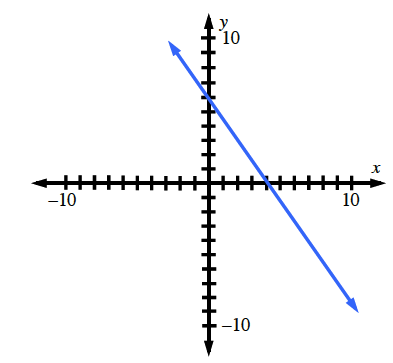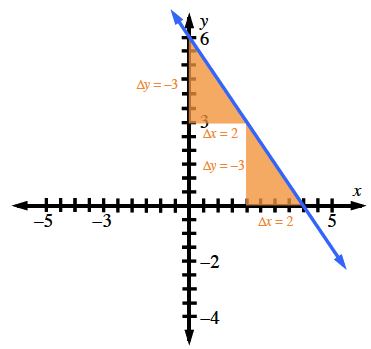### Home > CCG > Chapter 9 > Lesson 9.2.2 > Problem9-85

9-85.

On graph paper, graph the line $y=-\frac{3}{2}x+6$. State the $x\text{-}$ and $y\text{-intercepts}$.

$\text{Slope}=\frac{\Delta y}{\Delta x}=-\frac{3}{2}$

A good place to start: $(0,6)$.

Look at the graph. Where does the line cross the $x\text{-axis}$, and where does it cross the $y\text{-axis}$?